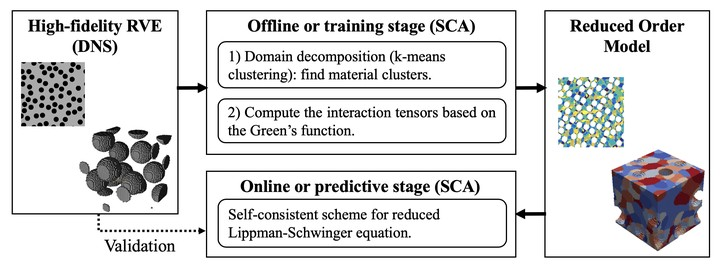# Self-consistent clustering analysisSCA framework

Self-consistent clustering analysis (SCA) is a reduced order model (ROM) technique first proposed in Liu et al. CMAME 2016. It enables a powerful tradeoff between efficiency and accuracy through synergistic exploitation of high-fidelity analysis and efficient mean field homogenization using a data-clustering technique.

SCA starts with an offline stage, in which a database is created by high-fidelity RVE analysis. A data compression algorithm (e.g. k-means clustering) is then used to establish clustering groups with similar mechanistic features such as the local strain concentration factor $\textbf{A}$:

$\boldsymbol{\varepsilon}^{\text{micro}}(\textbf{x})=\textbf{A}(\textbf{x}):\boldsymbol{\varepsilon}^{\text{macro}}.$

Material responses are assumed to be uniform in each cluster, and the interaction tensors between each pair of clusters $\textbf{D}^{IJ}$ are computed based on the Green’s function:

$\textbf{D}^{IJ}=\dfrac{1}{c^I\mid\Omega\mid}\int_{\Omega}\int_{\Omega} \chi^I(\textbf{x})\chi^J(\textbf{x}')\boldsymbol{\Phi}^0(\textbf{x},\textbf{x}')d\textbf{x}'d\textbf{x}.$

In the online stage, the discretized Lippmann-Schwinger integral equation is solved using a self-consistent scheme.

$\Delta\boldsymbol{\varepsilon}^I+\sum_{J=1}^{k}\textbf{D}^{IJ}:\left[\Delta\boldsymbol{\sigma}^J-\textbf{C}^0:\Delta\boldsymbol{\varepsilon}^J\right]-\Delta\boldsymbol{\varepsilon}^0=0.$

SCA has been applied to several material systems, including particle-reinforced composite, uni-directional fiber composite and polycrystalline materials. On-going work put more emphasis on new schemes to treat softening material with damage acrossing different scales. SCA has also been coupled with macroscopic finite element model to perform the so-called "multiscale concurrent simulations".